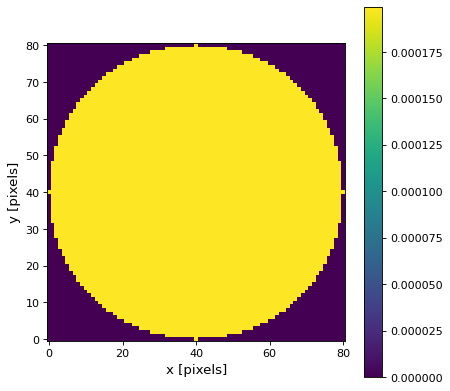# Tophat2DKernel¶

class `astropy.convolution.``Tophat2DKernel`(radius, **kwargs)[source]

2D Tophat filter kernel.

The Tophat filter is an isotropic smoothing filter. It can produce artifacts when applied repeatedly on the same data.

The generated kernel is normalized so that it integrates to 1.

Parameters
radius`int`

mode`str`, optional
One of the following discretization modes:
• ‘center’ (default)

Discretize model by taking the value at the center of the bin.

• ‘linear_interp’

Discretize model by performing a bilinear interpolation between the values at the corners of the bin.

• ‘oversample’

Discretize model by taking the average on an oversampled grid.

• ‘integrate’

Discretize model by integrating the model over the bin.

factornumber, optional

Factor of oversampling. Default factor = 10.

Examples

Kernel response:

```import matplotlib.pyplot as plt
from astropy.convolution import Tophat2DKernel
tophat_2D_kernel = Tophat2DKernel(40)
plt.imshow(tophat_2D_kernel, interpolation='none', origin='lower')
plt.xlabel('x [pixels]')
plt.ylabel('y [pixels]')
plt.colorbar()
plt.show()
```

(png, svg, pdf)